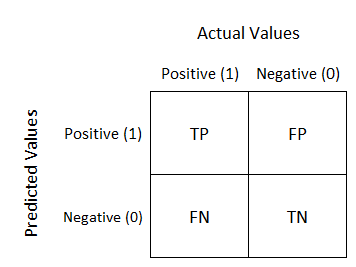# [Pytorch] Performance Evaluation of a Classification Model-Confusion Matrix

Original article was published by Yeseul Lee on Deep Learning on Medium# [Pytorch] Performance Evaluation of a Classification Model-Confusion Matrix

There are several ways to evaluate the performance of a classification model. One of them is a ‘Confusion Matrix’ which classifies our predictions into several groups depending on the model’s prediction and its actual class. Through calculating confusion matrix, we can get the model’s accuracy, sensitivity, specificity, positive predictive value(PPV), negative predictive value(NPV) and F1 score, which are useful performance indicators of the classifier.

This is the example confusion matrix(2*2) of a binary classifier. (If the number of model’s classes is n, shape of the confusion matrix is n*n)

Let’s define some basic terminologies.

• True Positive(TP): The model predicted ‘Positive’ and it’s actual class is ‘Positive’, which is ‘True’
• False Positive(FP): The model predicted ‘Positive’ and it’s actual class is ‘Negative’, which is ‘False’
• False Negative(FN): The model predicted ‘Negative’ and it’s actual class is ‘Positive’, which is ‘False’
• True Negative(TN): The model predicted ‘Negative’ and it’s actual class is ‘Negative’, which is ‘True’

These are the performance criteria calculated from the confusion matrix.

(P=TP+FN, N=TN+FP)

• Accuracy: (TP+TN)/(P+N)
• Sensitivity: TP/P
• Specificity: TN/N
• PPV: TP/(TP+FP)
• NPV: TN/(TN+FN)
• F1 score: 2*(PPV*Sensitivity)/(PPV+Sensitivity) =(2*TP)/(2*TP+FP+FN)

Then, there’s Pytorch codes to calculate confusion matrix and its accuracy, sensitivity, specificity, PPV and NPV.

`def getConfusionMatrix(model, show_image=False):    model.eval() #set the model to evaluation mode    confusion_matrix=np.zeros((2,2),dtype=int) #initialize a confusion matrix    num_images=testset_sizes['test'] #size of the testset    with torch.no_grad(): #disable back prop to test the model        for i, (inputs, labels) in enumerate(testloaders['test']):            inputs = inputs.to(device)            labels = labels.to(device)            #get predictions of the model            outputs = model(inputs)             _, preds = torch.max(outputs, 1)             #get confusion matrix            for j in range(inputs.size()):                 if preds[j]==1 and labels[j]==1:                    term='TP'                    confusion_matrix+=1                elif preds[j]==1 and labels[j]==0:                    term='FP'                    confusion_matrix+=1                elif preds[j]==0 and labels[j]==1:                    term='FN'                    confusion_matrix+=1                elif preds[j]==0 and labels[j]==0:                    term='TN'                    confusion_matrix+=1                #show image and its class in confusion matrix                    if show_image:                    print('predicted: {}'.format(class_names[preds[j]]))                    print(term)                    imshow(inputs.cpu().data[j])                    print()        #print results        print('Confusion Matrix: ')        print(confusion_matrix)        print()        print('Sensitivity: ', 100*confusion_matrix/(confusion_matrix+confusion_matrix))        print('Specificity: ', 100*confusion_matrix/(confusion_matrix+confusion_matrix))        print('PPV: ', 100*confusion_matrix/(confusion_matrix+confusion_matrix))        print('NPV: ', 100*confusion_matrix/(confusion_matrix+confusion_matrix))        return confusion_matrix`